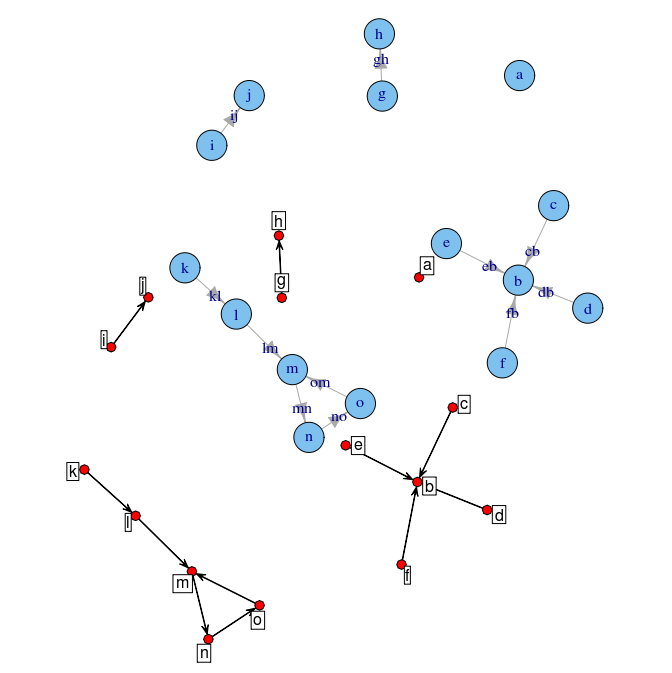Want to share your content on R-bloggers? click here if you have a blog, or here if you don't.

After some e-mails I decided to put together a bit more detailed instruction how to install the ‘intergraph’ package together with the namespaced version of the ‘network’ package. See here.

There seemed to be some problems with downloading the namespaced ‘network’ from my webstite (thanks Neil!). File permissions were set incorrectly. I fixed that and everything should be OK now.

Because it’s Friday, another example of using ‘intergraph’. This time plot a network using both ‘igraph’ and ‘network’ functionality on the same plotting device. Here is the result:And here is the code to produce it. `lx` in line 12  is “Deus Ex Machina”, or rather “Deus Ex locator()”…```# rescale 'x' to [-1; 1]
res <- function(x)
{
a <- -1 - (2*min(x))/(max(x)-min(x))
b <- 2 / (max(x) - min(x))
a + b*x
}
op <- par(mar=rep(0.5,4))
net <- as.network(exIgraph) # using 'intergraph'
set.seed(12345)
k <- apply(layout.fruchterman.reingold(exIgraph), 2, res)
lx <- c(-0.5, -1)
k2 <- sweep(k, 2, lx, "+")
plot(exIgraph, layout=k, rescale=FALSE, xlim=range(rbind(k, k2)[,1]), ylim=range(rbind(k, k2)[,2]) )
plot(net, coord=k2, displaylabels=TRUE, label=net %v% "label", new=FALSE)
par(op)
```

Filed under: networks, R, Uncategorized